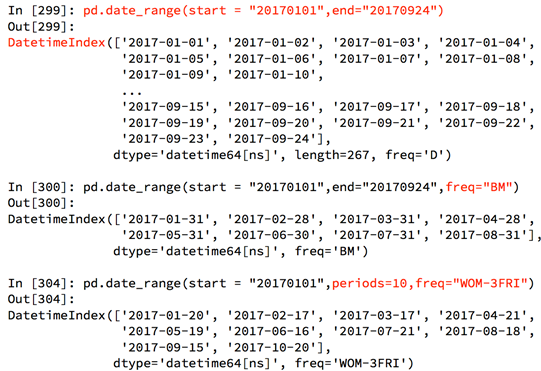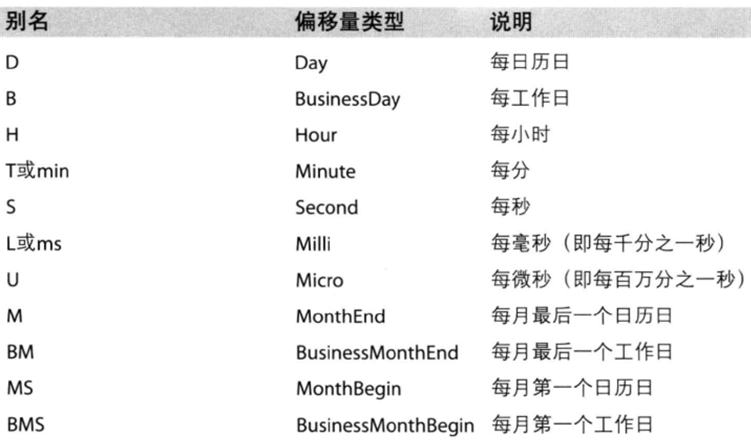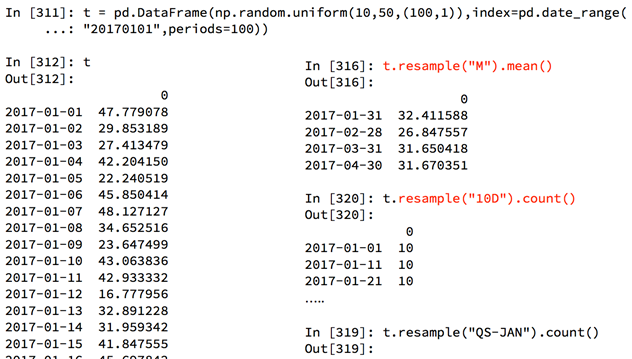机器学习-数据科学库（HM）_第6节_时间序列1389-李同学

时间序列

为什么要学习pandas中的时间序列

• pandas中处理时间序列非常简单
• 很多统计数据以及数据的规律都和时间序列有着非常重要的联系

生成一段时间范围

• pd.date_range(start=None, end=None, periods=None, freq=‘D’)
• start和end以及freq配合能够生成start和end范围内以频率freq的一组时间索引
• start和periods以及freq配合能够生成从start开始的频率为freq的periods个时间索引关于频率的更多缩写• 每三天写作3D，其他的频率也可以这样使用。

在DataFrame中使用时间序列

• 如果如上所示生成了一段时间范围，则将其设置为index
• index=pd.date_range(“20170101”,periods=10)
• df = pd.DataFrame(np.random.rand(10),index=index)
• 如果数据内有时间字符串，则使用pandas将其转化为时间序列：
• df[“timeStamp”] = pd.to_datetime(df[“timeStamp”], format="")
• format参数大部分情况下可以不用写，但是对于pandas无法格式化的时间字符串，我们可以使用该参数，比如包含中文

pandas重采样

• 重采样：指的是将时间序列从一个频率转化为另一个频率进行处理的过程
• 降采样：将高频率数据转化为低频率数据
• 升采样：低频率转化为高频率数据
• 在pandas中可以使用df.resample(“freq”)来实现这个需求应用：不同月份不同类型紧急电话的次数的变化情况（from ch5）

• 现在我们有2015到2017年25万条911的紧急电话的数据
• 统计出911数据中不同月份电话次数的变化情况
from matplotlib import pyplot as plt

# 把时间字符串转为时间类型，然后设置为索引
df["timeStamp"] = pd.to_datetime(df["timeStamp"])
df.set_index("timeStamp", inplace=True)

# 统计出911数据中不同月份的电话次数
count_by_month = df.resample("M").count()["title"]

# 画图
_x = count_by_month.index
_x = [i.strftime("%Y%m%d") for i in _x]	# 去掉时间戳后面多余的00:00:00
_y = count_by_month.values

plt.figure(figuresize=(20, 8), dpi=80)
plt.plot(range(len(_x)), _y)
plt.xticks(range(len(_x)), _x, rotation=45)
plt.show()– 统计出911数据中不同月份不同类型的电话的次数的变化情况

import pandas as pd
import numpy as np
from matplotlib import pyplot as plt

# 把时间字符串转为时间类型，然后设置为索引
df["timeStamp"] = pd.to_datetime(df["timeStamp"])

# 添加列，表示分类
temp_list = df["title"].str.split(": ").tolist()
cate_list = [i for i in temp_list]
df["cate"] = pd.DataFrame(np.array(cate_list).reshape((df.shape, 1)))

# 需要放到“添加列，表示分类”section之后，否则设置df["cate"]时二者的index不一样，cate列值会变为NaN
df.set_index("timeStamp", inplace=True)

plt.figure(figuresize=(20, 8), dpi=80)

# 分组
for group_name, group_data in df.groupby(by="cate"):
# 对不同的分类都进行绘图
# 统计出911数据中不同月份的电话次数
count_by_month = df.resample("M").count()["title"]

# 画图
_x = count_by_month.index
_x = [i.strftime("%Y%m%d") for i in _x]	# 去掉时间戳后面多余的00:00:00
_y = count_by_month.values

plt.plot(range(len(_x)), _y, label=group_name)

plt.xticks(range(len(_x)), _x, rotation=45)
plt.legend(loc="best")
plt.show()PeriodIndex

• DatetimeIndex：时间戳
• PeriodIndex：时间段
• 如果数据中给的时间信息是这个样子的• 则可使用以下方法，将其转化为pandas的时间类型
period = pd.PeriodIndex(year=df["year"], month=df["month'], day=df["day"], hour=df["hour"], freq=H)
df["datetime"] = period应用：PM2.5案例

• 现在我们有北上广、深圳、和沈阳5个城市空气质量数据，请绘制出5个城市的PM2.5随时间的变化情况
• 需要将时间使用PeriodIndex来转换
# coding=utf-8
import pandas as pd
from matplotlib import pyplot as plt

file_path = "./PM2.5/BeijingPm20100101_20151231.csv"

# 把分开的时间字符串通过PeriodINdex的方法转化为pandas的事件类型
period = pd.PeriodIndex(year=df["year"], month=df["month'], day=df["day"], hour=df["hour"], freq=H)
df["datetime"] = period

# 把datetime设置为索引
df.set_index("datetime", inplace=True)

# 进行降采样，取出每个月PM2.5的平均值
df = df.resample("7D").mean()

# 处理缺失数据，删除
data = df["PM_US Post"].dropna()
data_china = df["PM_ Dongsi"].dropna()

# 画图
_x = data.index
_x = [i.strftime("%Y%m%d") for i in _x]
_x_china = [i.strftime("%Y%m%d") for i in data_china.index]

_y = data.values
_y_china = data_china.values

plt.figure(figuresize=(20, 8), dpi=80)
plt.plot(range(len(_x)), _y, lable="US_POST")
plt.plot(range(len(_x_china)), _y_china, lable="CN_POST")
plt.xticks(range(0, len(_x), 10), list(_x)[::10], rotation=45)
plt.legend(loc="best")
plt.show()

评论 抢沙发觉得文章有用就打赏一下文章作者

支付宝扫一扫打赏微信扫一扫打赏Vieu3.3主题Q Q 登 录